Next: What is the face Up: Convex Polyhedron Previous: What is convex polytope/polyhedron?   Contents

## What are the faces of a convex polytope/polyhedron?

Let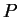be a convex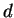-polyhedron (or-polytope) in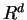.

For a real-vector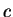and a real number, a linear inequality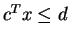is called valid forifholds for all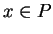. A subset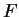of a polyhedronis called a face ofif it is represented as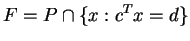for some valid inequality. By this definition, both the empty set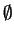and the whole setare faces. These two faces are called improper faces while the other faces are called proper faces.

We can define faces geometrically. For this, we need to define the notion of supporting hyperplanes. A hyperplane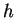ofis supportingif one of the two closed halfspaces ofcontains. A subsetofis called a face ofif it is either,itself or the intersection ofwith a supporting hyperplane.

The faces of dimension 0,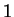,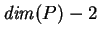and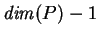are called the vertices, edges, ridges and facets, respectively. The vertices coincide with the extreme points ofwhich are defined as points which cannot be represented as convex combinations of two other points in. When an edge is not bounded, there are two cases: either it is a line or a half-line starting from a vertex. A half-line edge is called an extreme ray.Next: What is the face Up: Convex Polyhedron Previous: What is convex polytope/polyhedron?   Contents
Komei Fukuda 2004-08-26